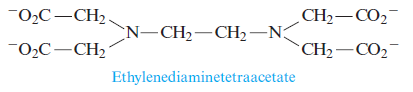# Problem: Calculate the concentration of Pb2+ in the following.c. Ethylenediaminetetraacetate (EDTA4-) is used as a complexing agent in chemical analysis and has the following structure:Solutions of EDTA4- are used to treat heavy metal poisoning by removing the heavy metal in the form of a soluble complex ion. The reaction of EDTA4- with Pb2+ isPb2+(aq) + EDTA4-(aq) ⇌ PbEDTA2-(aq)    K = 1.1 X 10 18Consider a solution with 0.010 mole of Pb(NO3)2 added to 1.0 L of an aqueous solution buffered at pH = 13.00 and containing 0.050 M Na4EDTA. Does Pb(OH)2 precipitate from this solution?

83% (10 ratings)
###### Problem Details

Calculate the concentration of Pb2+ in the following.

c. Ethylenediaminetetraacetate (EDTA4-) is used as a complexing agent in chemical analysis and has the following structure:Solutions of EDTA4- are used to treat heavy metal poisoning by removing the heavy metal in the form of a soluble complex ion. The reaction of EDTA4- with Pb2+ is

Pb2+(aq) + EDTA4-(aq) ⇌ PbEDTA2-(aq)    K = 1.1 X 10 18

Consider a solution with 0.010 mole of Pb(NO3)2 added to 1.0 L of an aqueous solution buffered at pH = 13.00 and containing 0.050 M Na4EDTA. Does Pb(OH)2 precipitate from this solution?